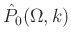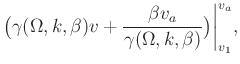## Analytical path-summation migration

Landa et al. (2006) present a path-integral imaging approach, which does not require knowledge of a velocity distribution in the subsurface. Burnett et al. (2011) adopt this approach and employ the principle of diffraction apex stationarity under migration velocity perturbation (Novais et al., 2006) to evaluate the diffraction path-summation migration integralthrough stacking of constant migration velocity images. The images are generated by velocity continuation (VC) technique, which corresponds to the following transformation in the Fourier domain (Fomel, 2003):(1)

whereis VC velocity,is the Fourier dual of(time axis after the transform),is the wavenumber;is input stacked and not migrated data, which corresponds toandis a constant velocity image.

In our previous work (Merzlikin and Fomel, 2017a), we derived analytical formulae for path-summation integral evaluation based on the continuity of VC transformation with respect to velocity: stacking of constant migration velocity images is replaced by a direct integral expression. Analytical formulae allow us to treat path-summation imaging workflow as a simple filter in the frequency-wavenumber domain. Here, we extend Gaussian weighting path-summation migration scheme (Merzlikin and Fomel, 2017a) to account for variable velocity in the subsurface: we design a weighting function, which has a flat region in the middle and has a Gaussian decay for the tail-elimination region as opposed to a curve with one most probable velocity value in regular Gaussian weighting path-summation integral. The following expression describes path-summation integral with Gaussian tapering:(2)

whereandcorrespond to the lowest and to the highest constant migration velocities in the image set being stacked or, precisely speaking, integrated;andare tail cut-off velocities, which correspond to the slope decay parameter. Expression 2 corresponds to the sum of three path-summation integrals: two Gaussian weighting scheme path-summation integrals (the first and the last terms) and unweighted path-summation integral (the middle term). Each term has an analytical expression. Gaussian weighting scheme path-summation integrals can be computed as (Merzlikin and Fomel, 2017a):erfi(3)

where. To compute the last summation term, which corresponds to the right taper slope, we replacewithandwith. The middle term can be evaluated as:erfi(4)

Thus, Gaussian-tapering path-summation integral can be evaluated analytically with a cost equal to that of only two FFTs.

2019-07-17